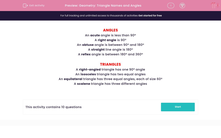# Geometry: Triangle Names and Angles

In this worksheet, students name the different types of angles and triangles.Key stage:  KS 2

Curriculum topic:   Maths and Numerical Reasoning

Difficulty level:#### Worksheet Overview

ANGLES

An acute angle is less than 90º

right angle is 90º

An obtuse angle is between 90º and 180º

straight line angle is 180º

reflex angle is between 180º and 360º

TRIANGLES

A right-angled triangle has one 90º angle

An isosceles triangle has two equal angles

An equilateral triangle has three equal angles, each of size 60º

A scalene triangle has three different angles

### What is EdPlace?

We're your National Curriculum aligned online education content provider helping each child succeed in English, maths and science from year 1 to GCSE. With an EdPlace account you’ll be able to track and measure progress, helping each child achieve their best. We build confidence and attainment by personalising each child’s learning at a level that suits them.

Get started